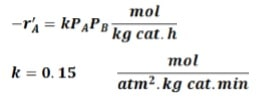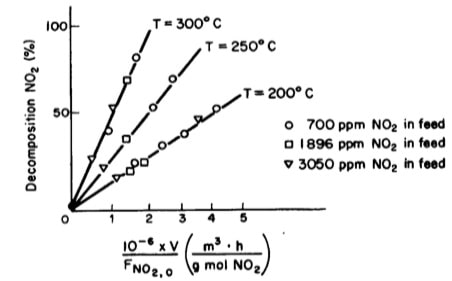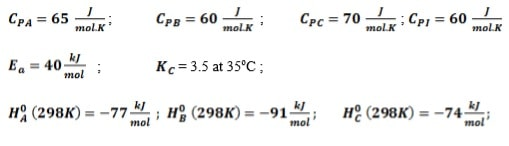# Reactor Technology IV Assignment

```{`VAAL UNIVERSITY OF TECHNOLOGY
DEPARTMENT OF CHEMICAL ENGINEERING
SUBJECT: REACTOR TECHNOLOGY IV
SUBJECT CODE: EHTRN4A
`}```

## Question 1 Marks 20

Ethane is pyrolysed in a plug flow reactor at 815°C and 2.87 atm:

C2H6 ⇔ C2H4 + H2

At the reaction conditions equilibrium is represented by Kp = PPBPAC =1.45atm

Feed to the reactor is pure ethane. Calculate the molar feed rate of ethane that would be required to achieve 90% of the equilibrium conversion in a 100 dm3 reactor. k = 1.1 min-1 at 815°C.

## Question 2 Marks 20

The following reaction is taking place in a PBR: A + B {`->`} 2C

The feed consists of 15% inerts with the remainder being a stoichiometric mixture of the 2 reactants. The feed enters the reactor at the rate of 1.5 kmol/h at 260 oC and 2 atm, the pressure drop parameter 𝛼?=0.004 𝑘𝑔−1. The reaction rate and the rate constant are given by:Calculate the catalyst weight required to achieve 75% conversion for the following cases:

1. In the absence of pressure drop

In the presence of pressure drop

## Question 3 Marks 20

The elementary, liquid-phase, irreversible reaction A+BC is is to be carried out in a flow reactor. Two reactors are available, an 800 dm3 PFR that can only be operated at 300 K and a 200 dm3 CSTR that can be operated at 350 K. The two feed streams to the reactor mix to form a single feed stream that is equal molar in A and B, with a total volumetric flowrate of 10 dm3/min. Which of the two reactors will give us the highest conversion?

• At 300 K, k = 0.07 dm3/mol-min
• E = 85000 J/mol-K
• CA0 before mixing = CB0 before mixing = 2 mol/dm3
• vA0 = vB0 = 0.5*v0 = 5 dm3/min

## Question 4 Marks 20

A reactor bomb fitted to a sensitive pressure measuring device is filled with a gas containing 40% A and 60 % inerts to a total pressure of 4 atm at 25oC. The temperature of the bomb was rapidly raised to 100oC. At this temperature, A decomposes according to the stoichiometry:

A → 2B

 Time (min) 1 2 4 6 8 10 12 Total pressure in reactor at 100oC 5.19 5.36 5.66 5.9 6.1 6.26 6.4

Assume there is no significant reaction at 25oC and during the rise to 100oC, determine the rate law of decomposition of A.

## Question 5 Marks 15

Tests were run on a small experimental reactor used for decomposing nitrogen oxides in an automobile exhaust stream. In one series of tests, a nitrogen stream containing various concentrations of NO2 was fed to a reactor and the kinetic data obtained is shown in figure 1 below. Each point represents one complete run. The reactor operates essentially as an isothermal backmix reactor (CSTR).

1. What can you deduce about the apparent order of the reaction over the temperature range studied?
2. What is the specific rate of reaction for the test conducted at 300oC.xFigure1: Plot of percent decomposition of NO2 V/FAO

## Question 6 Marks 25

The following elementary reversible gas phase reaction is carried out adiabatically in a PFR. 2A ⇔ B + C

A feed containing 75% A and 25 % inert enters the reactor at the rate of 120 kmol/h, temperature of 310K and pressure of 10 atm. The specific reaction rate at 325K is 0.5 dm3.mol-1.s-1. Assuming the pressure drop is negligible:

1. Determine the expression for equilibrium conversion
2. By making use of Simpson’s three-eights rule, determine the volume necessary to achieve 72% conversion of A
3. Determine the maximum conversion that can be achieved for this exothermic reaction as well as the adiabatic temperature